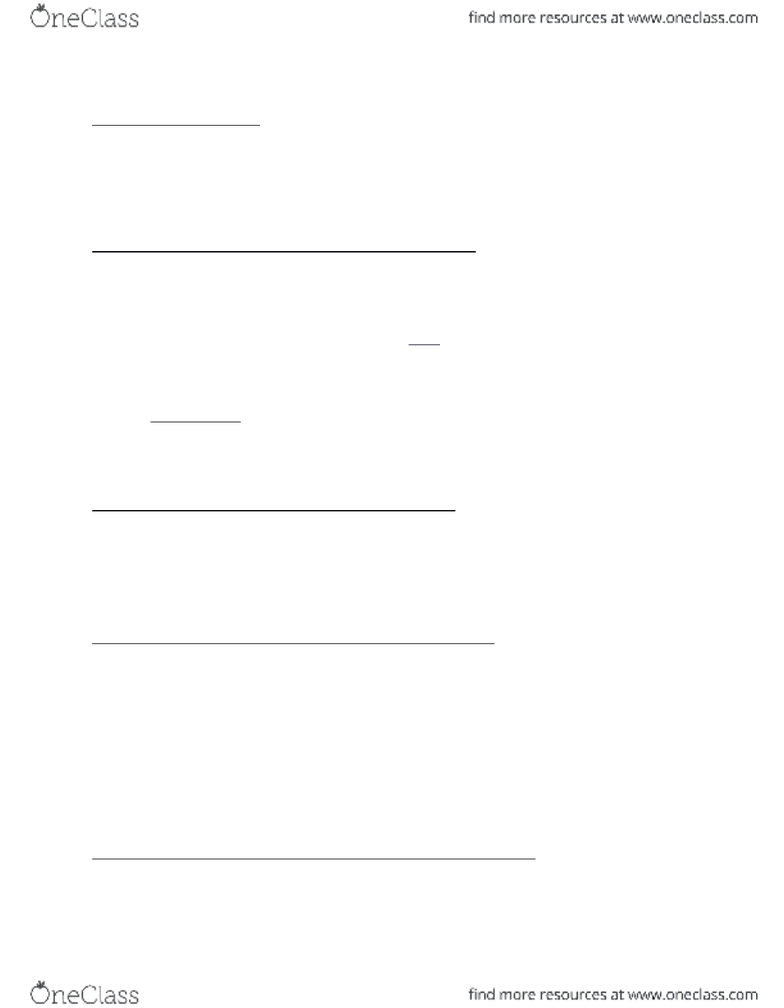Textbook Notes (270,000)
CA (160,000)
UTSC (20,000)
Iris Au (30)
Chapter 12

# MGEA06H3 Chapter Notes - Chapter 12: Price Level, Aggregate Demand, Aggregate Supply

Department
Economics for Management Studies
Course Code
MGEA06H3
Professor
Iris Au
Chapter
12

This preview shows half of the first page. to view the full 3 pages of the document.Macroeconomics Notes: Lecture Six (Chapter Twelve) Part One and Two:
Aggregate Demand (AD):
This shows the relationship between the aggregate price level and the quantity o
aggregate output demanded by household’s business the government and the rest of the
world.
Holding everything constant, there’s an inverse relationship between the aggregate price
level (P) and the quantity of aggregate output demanded.
Why Is the Aggregate Demand Curve Downward Sloping?
GDP = Y = C + I + G + X – IM
The demand curve for individual goods shows how the quantity demanded depends on
the price of that good.
The quantity demand falls when the price of that good rises.
In macroeconomics, we look at the demand for ALL goods and services.
If all prices, including prices of labour & capital, increase, then dollar incomes also
increase.
If both incomes and prices increase at the same rate, there is no change in real income =
Dollar income
Price
there should be no change in aggregate demand.
A downward sloping AD curve can be explained by the wealth effect and the interest rate
effect.
The Wealth Effect of an Aggregate Price Level Change:
This is the effect on consumer spending caused by the effect of a change in the aggregate
price level on the purchasing power of consumer’s assets.
Holding all else constant, a rise in price lowers the real value of existing assets real value
of wealth .
When P , wealth (autonomous) C (Chapters 10 & 11) AD .
The Interest Rate Effect of an Aggregate Price Level Change:
This is the effect on consumer spending and investment spending caused by the effect of a
change in the aggregate price level on the purchasing power of consumer and firms money
holding.
When interest rate , cost of borrowing investment . (Chs 10 & 11)
When interest rate , current consumption become more expensive consumption .
(Chapter 10)
An open economy will also experience (net) capital inflows when interest rate ↑⇒ domestic
currency appreciates X & IM NX . (Ch 19)
In conclusion, C , I & NX when price AD .
The Aggregate Demand Curve and the Income-Expenditure Model:
To derive the AD curve from the income-expenditure model, we need to take the effect of a
price change on planned aggregate expenditure into account:
Along an AEPlanned line, we hold the aggregate price level fixed.
###### You're Reading a Preview

Unlock to view full version